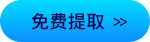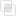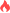python里的π怎么输入

Python2021-04-28 09:49:08510浏览 · 0收藏 · 0评论1、说明

python中的π是math.pi变量。它包含在标准库中。在试图计算它之前，应该先导入math库，然后再调用math.pi方法。

2、实例

```def main():

a=1.0

b=1.0/math.sqrt(2)

t=1.0/4.0

p=1.0

for i in range(1000):

at=(a+b)/2

bt=math.sqrt(a*b)

tt=t-p*(a-at)**2

pt=2*p

a=at;b=bt;t=tt;p=pt

my_pi=(a+b)**2/(4*t)

print("Pi is approximately: "+str(my_pi))

if __name__== "__main__":

main()```python使用items()遍历键值对python函数如何指定默认值python函数中返回值的作用python在函数中传递实参python使用import导入导出python使用as指定别名python编写函数的注意点python类属性设置默认值python修改属性值有哪些方法python导入类的不同方式python如何读取全部的文件python json保存数据的方法Python找回文子串的方法java反射调用指定的属性258

java获取class实例的方式636

java中反射提供哪些功能867